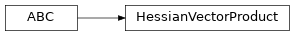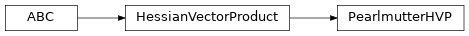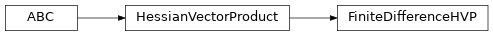# garage.tf.optimizers.conjugate_gradient_optimizer¶

Computes the decent direction using the conjugate gradient method, and then computes the optimal step size that will satisfy the KL divergence constraint. Finally, it performs a backtracking line search to optimize the objective.

class HessianVectorProduct(num_slices=1)

Bases: abc.ABCBase class for computing Hessian-vector product.

Parameters

num_slices (int) – Hessian-vector product function’s inputs will be divided into num_slices and then averaged together to improve performance.

abstract update_hvp(self, f, target, inputs, reg_coeff, name=None)

Build the symbolic graph to compute the Hessian-vector product.

Parameters
• f (tf.Tensor) – The function whose Hessian needs to be computed.

• target (garage.tf.policies.Policy) – A parameterized object to optimize over.

• inputs (tuple[tf.Tensor]) – The inputs for function f.

• reg_coeff (float) – A small value so that A -> A + reg*I.

• name (str) – Name to be used in tf.name_scope.

build_eval(self, inputs)

Build the evaluation function. # noqa: D202, E501 # https://github.com/PyCQA/pydocstyle/pull/395.

Parameters

inputs (tuple[numpy.ndarray]) – Function f will be evaluated on these inputs.

Returns

It can be called to get the final result.

Return type

function

class PearlmutterHVP(num_slices=1)Computes Hessian-vector product using Pearlmutter’s algorithm.

Pearlmutter, Barak A. “Fast exact multiplication by the Hessian.” Neural

computation 6.1 (1994): 147-160.

update_hvp(self, f, target, inputs, reg_coeff, name='PearlmutterHVP')

Build the symbolic graph to compute the Hessian-vector product.

Parameters
• f (tf.Tensor) – The function whose Hessian needs to be computed.

• target (garage.tf.policies.Policy) – A parameterized object to optimize over.

• inputs (tuple[tf.Tensor]) – The inputs for function f.

• reg_coeff (float) – A small value so that A -> A + reg*I.

• name (str) – Name to be used in tf.name_scope.

build_eval(self, inputs)

Build the evaluation function. # noqa: D202, E501 # https://github.com/PyCQA/pydocstyle/pull/395.

Parameters

inputs (tuple[numpy.ndarray]) – Function f will be evaluated on these inputs.

Returns

It can be called to get the final result.

Return type

function

class FiniteDifferenceHVP(base_eps=1e-08, symmetric=True, num_slices=1)Computes Hessian-vector product using finite difference method.

Parameters
• base_eps (float) – Base epsilon value.

• symmetric (bool) – Symmetric or not.

• num_slices (int) – Hessian-vector product function’s inputs will be divided into num_slices and then averaged together to improve performance.

update_hvp(self, f, target, inputs, reg_coeff, name='FiniteDifferenceHVP')

Build the symbolic graph to compute the Hessian-vector product.

Parameters
• f (tf.Tensor) – The function whose Hessian needs to be computed.

• target (garage.tf.policies.Policy) – A parameterized object to optimize over.

• inputs (tuple[tf.Tensor]) – The inputs for function f.

• reg_coeff (float) – A small value so that A -> A + reg*I.

• name (str) – Name to be used in tf.name_scope.

build_eval(self, inputs)

Build the evaluation function. # noqa: D202, E501 # https://github.com/PyCQA/pydocstyle/pull/395.

Parameters

inputs (tuple[numpy.ndarray]) – Function f will be evaluated on these inputs.

Returns

It can be called to get the final result.

Return type

function

class ConjugateGradientOptimizer(cg_iters=10, reg_coeff=1e-05, subsample_factor=1.0, backtrack_ratio=0.8, max_backtracks=15, accept_violation=False, hvp_approach=None, num_slices=1)

Performs constrained optimization via line search.

The search direction is computed using a conjugate gradient algorithm, which gives x = A^{-1}g, where A is a second order approximation of the constraint and g is the gradient of the loss function.

Parameters
• cg_iters (int) – The number of CG iterations used to calculate A^-1 g

• reg_coeff (float) – A small value so that A -> A + reg*I

• subsample_factor (float) – Subsampling factor to reduce samples when using “conjugate gradient. Since the computation time for the descent direction dominates, this can greatly reduce the overall computation time.

• backtrack_ratio (float) – backtrack ratio for backtracking line search.

• max_backtracks (int) – Max number of iterations for backtrack linesearch.

• accept_violation (bool) – whether to accept the descent step if it violates the line search condition after exhausting all backtracking budgets.

• hvp_approach (HessianVectorProduct) – A class that computes Hessian-Vector products.

• num_slices (int) – Hessian-vector product function’s inputs will be divided into num_slices and then averaged together to improve performance.

update_opt(self, loss, target, leq_constraint, inputs, extra_inputs=None, name='ConjugateGradientOptimizer', constraint_name='constraint')

Update the optimizer.

Build the functions for computing loss, gradient, and the constraint value.

Parameters
• loss (tf.Tensor) – Symbolic expression for the loss function.

• target (garage.tf.policies.Policy) – A parameterized object to optimize over.

• leq_constraint (tuple[tf.Tensor, float]) – A constraint provided as a tuple (f, epsilon), of the form f(*inputs) <= epsilon.

• inputs (list(tf.Tenosr)) – A list of symbolic variables as inputs, which could be subsampled if needed. It is assumed that the first dimension of these inputs should correspond to the number of data points.

• extra_inputs (list[tf.Tenosr]) – A list of symbolic variables as extra inputs which should not be subsampled.

• name (str) – Name to be passed to tf.name_scope.

• constraint_name (str) – A constraint name for prupose of logging and variable names.

loss(self, inputs, extra_inputs=None)

Compute the loss value.

Parameters
• inputs (list[numpy.ndarray]) – A list inputs, which could be subsampled if needed. It is assumed that the first dimension of these inputs should correspond to the number of data points

• extra_inputs (list[numpy.ndarray]) – A list of extra inputs which should not be subsampled.

Returns

Loss value.

Return type

float

constraint_val(self, inputs, extra_inputs=None)

Constraint value.

Parameters
• inputs (list[numpy.ndarray]) – A list inputs, which could be subsampled if needed. It is assumed that the first dimension of these inputs should correspond to the number of data points

• extra_inputs (list[numpy.ndarray]) – A list of extra inputs which should not be subsampled.

Returns

Constraint value.

Return type

float

optimize(self, inputs, extra_inputs=None, subsample_grouped_inputs=None, name='optimize')

Optimize the function.

Parameters
• inputs (list[numpy.ndarray]) – A list inputs, which could be subsampled if needed. It is assumed that the first dimension of these inputs should correspond to the number of data points

• extra_inputs (list[numpy.ndarray]) – A list of extra inputs which should not be subsampled.

• subsample_grouped_inputs (list[numpy.ndarray]) – Subsampled inputs to be used when subsample_factor is less than one.

• name (str) – The name argument for tf.name_scope.